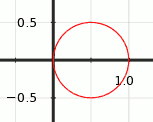# Maximum Distance Within a Circle

#### Mathsnut

##### New member
If lines L1 & L2 form a right angle within a circle, at what point P are the lengths of L1 + L2 maximized?

Note: the right angle is not bound to the circumference, but may be placed at any location within the circle.

#### HallsofIvy

##### Elite Member
What points are you taking as the "starting" and "ending" points of the line segments? If the lines start and end on the circle then obviously the lines will be longest if they are diameters of the circle so P is the center of the circle.

#### Cubist

##### Junior Member
•Mathsnut

#### lev888

##### Full Member
If lines L1 & L2 form a right angle within a circle, at what point P are the lengths of L1 + L2 maximized?

Note: the right angle is not bound to the circumference, but may be placed at any location within the circle.
I think the idea is to find not the point, but how the lines are oriented relative to the center of the circle.
Pick a point P on the circumference. Draw the 2 lines. Start turning the lines around P. The angle of rotation is your variable. Figure out the length as a function of the angle. Find the maximum.

#### Cubist

##### Junior Member
It does seem a very good bet that P lies on the circumference. If you want to try lev888's suggestion, then I have a hint. There is a very simple polar coordinate equation that gives the following graph:-For −π/2 ≤ θ ≤ π/2 and r = ? < a trig function, do you know it? >

Can you see how this helps?

--

However I am also wondering if you are studying partial derivatives in class? If so, then your teacher probably wants you to solve this question in a different way (by considering ALL points within the circle).

•lev888